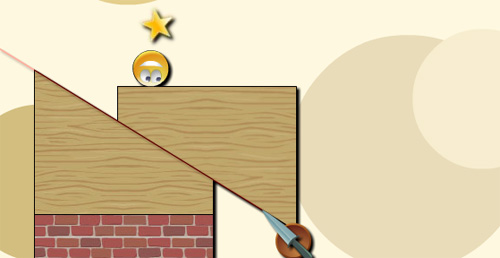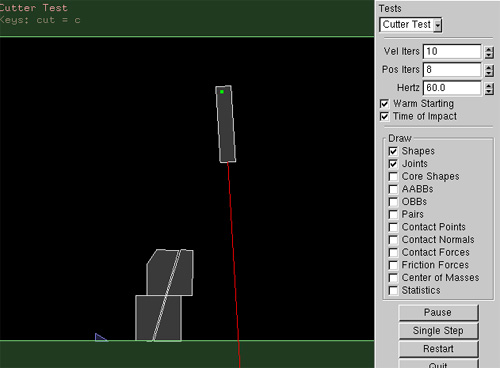# The engine behind Splitter Flash game

If you played Splitter in these days, you probably enjoyed the gameplay thanks to the knife cutting and slicing various objects in the gameObviously you can made your own splitter game with BOX2D, and the “only” problem seems to be the knife.

Well, in the BOX2D official forum there is a thread where a user created his own slicing engine (with a laser in the example) and released the source code.

This is what you will get with the script:The boxes have been cut by the laser, sorry for the picture but I was controlling the laser with the mouse, pressing “C” to cut and “Print” to capture the image… I wasn’t quick enough…

The source code is C, but you (or me) can easily port it into AS3.

```/*
* Copyright (c) 2007 Erin Catto http://www.gphysics.com
*
* This software is provided 'as-is', without any express or implied
* warranty.  In no event will the authors be held liable for any damages
* arising from the use of this software.
* Permission is granted to anyone to use this software for any purpose,
* including commercial applications, and to alter it and redistribute it
* freely, subject to the following restrictions:
* 1. The origin of this software must not be misrepresented; you must not
* claim that you wrote the original software. If you use this software
* in a product, an acknowledgment in the product documentation would be
* appreciated but is not required.
* 2. Altered source versions must be plainly marked as such, and must not be
* misrepresented as being the original software.
* 3. This notice may not be removed or altered from any source distribution.
*/

#ifndef CUTTER_TEST_H
#define CUTTER_TEST_H

class CutterTest : public Test
{
public:
CutterTest()
{
//m_world->SetGravity(b2Vec2(0,0));

b2Body* ground = NULL;
{
b2BodyDef bd;
bd.position.Set(0.0f, -10.0f);
ground = m_world->CreateBody(&bd);

b2PolygonDef sd;
sd.SetAsBox(50.0f, 10.0f);
ground->CreateShape(&sd);
}

{
b2BodyDef bd;
bd.position.Set(0.0f, 50.0f);
ground = m_world->CreateBody(&bd);

b2PolygonDef sd;
sd.SetAsBox(50.0f, 10.0f);
ground->CreateShape(&sd);
}

{
b2BodyDef bd;
bd.position.Set(0.0f, 1.0f);
laserBody = m_world->CreateBody(&bd);

b2PolygonDef sd;
sd.SetAsBox(5.0f, 1.0f);
sd.density = 4.0;
laserBody->CreateShape(&sd);
laserBody->SetMassFromShapes();
}

{
b2PolygonDef sd;
sd.SetAsBox(3.0f, 3.0f);
sd.density = 5.0f;

b2BodyDef bd;
bd.userData = (void*)1;
bd.position.Set(0.0f, 8.0f);
b2Body* body1 = m_world->CreateBody(&bd);
body1->CreateShape(&sd);
body1->SetMassFromShapes();
}

{
b2PolygonDef sd;
sd.SetAsBox(3.0f, 3.0f);
sd.density = 5.0f;

b2BodyDef bd;
bd.userData = (void*)1;
bd.position.Set(0.0f, 8.0f);
b2Body* body1 = m_world->CreateBody(&bd);
body1->CreateShape(&sd);
body1->SetMassFromShapes();
}
}

int CheckPolyShape(const b2PolygonDef* poly)
{
if (!(3 <= poly->vertexCount && poly->vertexCount <= b2_maxPolygonVertices))
return -1;

b2Vec2 m_normals[poly->vertexCount];

// Compute normals. Ensure the edges have non-zero length.
for (int32 i = 0; i < poly->vertexCount; ++i)
{
int32 i1 = i;
int32 i2 = i + 1 < poly->vertexCount ? i + 1 : 0;
b2Vec2 edge = poly->vertices[i2] - poly->vertices[i1];
if (!(edge.LengthSquared() > B2_FLT_EPSILON * B2_FLT_EPSILON))
return -1;
m_normals[i] = b2Cross(edge, 1.0f);
m_normals[i].Normalize();
}

// Ensure the polygon is convex.
for (int32 i = 0; i < poly->vertexCount; ++i)
{
for (int32 j = 0; j < poly->vertexCount; ++j)
{
// Don't check vertices on the current edge.
if (j == i || j == (i + 1) % poly->vertexCount)
{
continue;
}

// Your polygon is non-convex (it has an indentation).
// Or your polygon is too skinny.
float32 s = b2Dot(m_normals[i], poly->vertices[j] - poly->vertices[i]);
if (!(s < -b2_linearSlop))
return -1;
}
}

// Ensure the polygon is counter-clockwise.
for (int32 i = 1; i < poly->vertexCount; ++i)
{
float32 cross = b2Cross(m_normals[i-1], m_normals[i]);

// Keep asinf happy.
cross = b2Clamp(cross, -1.0f, 1.0f);

// You have consecutive edges that are almost parallel on your polygon.
float32 angle = asinf(cross);
if (!(angle > b2_angularSlop))
return -1;
}

// Compute the polygon centroid.
b2Vec2 m_centroid; m_centroid.Set(0.0f, 0.0f);
float32 area = 0.0f;

// pRef is the reference point for forming triangles.
// It's location doesn't change the result (except for rounding error).
b2Vec2 pRef(0.0f, 0.0f);

const float32 inv3 = 1.0f / 3.0f;

for (int32 i = 0; i < poly->vertexCount; ++i)
{
// Triangle vertices.
b2Vec2 p1 = pRef;
b2Vec2 p2 = poly->vertices[i];
b2Vec2 p3 = i + 1 < poly->vertexCount ? poly->vertices[i+1] : poly->vertices;

b2Vec2 e1 = p2 - p1;
b2Vec2 e2 = p3 - p1;

float32 D = b2Cross(e1, e2);

float32 triangleArea = 0.5f * D;
area += triangleArea;

// Area weighted centroid
m_centroid += triangleArea * inv3 * (p1 + p2 + p3);
}

// Centroid
if (!(area > B2_FLT_EPSILON))
return -1;
m_centroid *= 1.0f / area;

// Compute the oriented bounding box.
//ComputeOBB(&m_obb, m_vertices, m_vertexCount);

// Create core polygon shape by shifting edges inward.
// Also compute the min/max radius for CCD.
for (int32 i = 0; i < poly->vertexCount; ++i)
{
int32 i1 = i - 1 >= 0 ? i - 1 : poly->vertexCount - 1;
int32 i2 = i;

b2Vec2 n1 = m_normals[i1];
b2Vec2 n2 = m_normals[i2];
b2Vec2 v = poly->vertices[i] - m_centroid;

b2Vec2 d;
d.x = b2Dot(n1, v) - b2_toiSlop;
d.y = b2Dot(n2, v) - b2_toiSlop;

// Shifting the edge inward by b2_toiSlop should
// not cause the plane to pass the centroid.

if (!(d.x >= 0.0f))
return -1;
if (!(d.y >= 0.0f))
return -1;
}

return 0;
}

/// Split a shape trough a segment
///	@return
/// -1 - Error on split
///  0 - Normal result is two new shape definitions.
int SplitShape(b2PolygonShape* shape, const b2Segment& segment, float splitSize, b2PolygonDef* newPolygon)
{
assert(shape != NULL);
assert(newPolygon != NULL);
assert(splitSize >= 0);

float lambda = 1;
b2Vec2 normal;

const b2Body* b = shape->GetBody();
const b2XForm xf = b->GetXForm();
if (shape->TestSegment(xf, &lambda, &normal, segment, 1.0f) != e_hitCollide)
return -1;
b2Vec2 entryPoint = (1-lambda)*segment.p1+lambda*segment.p2;

b2Segment reverseSegment;
reverseSegment.p1 = segment.p2;
reverseSegment.p2 = segment.p1;

if (shape->TestSegment(xf, &lambda, &normal, reverseSegment, 1.0f) != e_hitCollide)
return -1;
b2Vec2 exitPoint = (1-lambda)*reverseSegment.p1+lambda*reverseSegment.p2;

b2Vec2 localEntryPoint = b->GetLocalPoint(entryPoint);
b2Vec2 localExitPoint = b->GetLocalPoint(exitPoint);
const b2Vec2* vertices = shape->GetVertices();
int last = -1;
for(int i=0;iGetVertexCount();i++)
{
int n;
//Find out if this vertex is on the old or new shape.
if (b2Dot(b2Cross(localExitPoint-localEntryPoint, 1), vertices[i]-localEntryPoint) > 0)
n = 0;
else
n = 1;
if (last != n)
{
//If we switch from one shape to the other add the cut vertices.
if (last == 0)
{
newPolygon[last].vertices[newPolygon[last].vertexCount] = localExitPoint;
newPolygon[last].vertexCount++;
newPolygon[last].vertices[newPolygon[last].vertexCount] = localEntryPoint;
newPolygon[last].vertexCount++;
}
if (last == 1)
{
newPolygon[last].vertices[newPolygon[last].vertexCount] = localEntryPoint;
newPolygon[last].vertexCount++;
newPolygon[last].vertices[newPolygon[last].vertexCount] = localExitPoint;
newPolygon[last].vertexCount++;
}
}
newPolygon[n].vertices[newPolygon[n].vertexCount] = vertices[i];
newPolygon[n].vertexCount++;
last = n;
}

{
newPolygon.vertices[newPolygon.vertexCount] = localExitPoint;
newPolygon.vertexCount++;
newPolygon.vertices[newPolygon.vertexCount] = localEntryPoint;
newPolygon.vertexCount++;
}
{
newPolygon.vertices[newPolygon.vertexCount] = localEntryPoint;
newPolygon.vertexCount++;
newPolygon.vertices[newPolygon.vertexCount] = localExitPoint;
newPolygon.vertexCount++;
}

for(int n=0;n<2;n++)
{
b2Vec2 offset;
{
}else{
offset = (newPolygon[n].vertices[newPolygon[n].vertexCount-1] - newPolygon[n].vertices);
}
offset.Normalize();

{
}else{
offset = (newPolygon[n].vertices - newPolygon[n].vertices[newPolygon[n].vertexCount-1]);
}
offset.Normalize();

}

/*
//Check if the new shapes are not too tiny. (TODO: still generates shapes which fail assert checks)
for(int n=0;n<2;n++)
for(int i=0;iGetWorldPoint(laserStart);
segment.p2 = segment.p1 + laserBody->GetWorldVector(laserDir);

const int max_shapes = 64;
b2Shape* shapes[max_shapes];
int count = m_world->Raycast(segment, shapes, max_shapes, false, NULL);
for(int i=0;iGetType() != e_polygonShape)
continue;

b2Body* b = shapes[i]->GetBody();
//Custom check to make sure we don't cut stuff we don't want to cut.
if (b->GetUserData() != (void*)1)
continue;//return if we cannot pass trough uncutable shapes.

b2PolygonShape* polyShape = (b2PolygonShape*)shapes[i];
b2PolygonDef pd;
pd.density = 5.0f;
pd.density = 5.0f;

if (SplitShape(polyShape, segment, 0.1, pd) == 0)
{
b->DestroyShape(shapes[i]);
b->CreateShape(&pd);
b->SetMassFromShapes();
b->WakeUp();

b2BodyDef bd;
bd.userData = (void*)1;
bd.position = b->GetPosition();
bd.angle = b->GetAngle();
b2Body* newBody = m_world->CreateBody(&bd);
newBody->CreateShape(&pd);
newBody->SetMassFromShapes();
newBody->SetAngularVelocity(b->GetAngularVelocity());
newBody->SetLinearVelocity(b->GetLinearVelocity());
}
}
}

void Keyboard(unsigned char key)
{
switch (key)
{
case 0:
break;
case 'c':
Cut();
break;
}
}

void Step(Settings* settings)
{
m_debugDraw.DrawString(5, m_textLine, "Keys: cut = c");
m_textLine += 15;

Test::Step(settings);

float32 segmentLength = 30.0f;

b2Segment segment;
b2Vec2 laserStart(5.0f-0.1f,0.0f);
b2Vec2 laserDir(segmentLength,0.0f);
segment.p1 = laserBody->GetWorldPoint(laserStart);
segment.p2 = laserBody->GetWorldVector(laserDir);
segment.p2+=segment.p1;

b2Color laserColor(1,0,0);
m_debugDraw.DrawSegment(segment.p1, segment.p2, laserColor);
}

static Test* Create()
{
return new CutterTest;
}

b2Body* laserBody;

};

#endif```

Fortune and fame in this blog for who will convert it, otherwise it will be me…

This is the source code.

214 GAME PROTOTYPES EXPLAINED WITH SOURCE CODE
// 1+2=3
// 10000000
// 2 Cars
// 2048
// Avoider
// Ballz
// Block it
// Blockage
// Bloons
// Boids
// Bombuzal
// Breakout
// Bricks
// Columns
// CubesOut
// Dots
// DROP'd
// Dudeski
// Eskiv
// Filler
// Fling
// Globe
// HookPod
// Hundreds
// InkTd
// Iromeku
// Lumines
// Magick
// MagOrMin
// Maze
// Memdot
// Nano War
// Nodes
// o:anquan
// Ononmin
// Pacco
// Phyballs
// Platform
// Poker
// Pool
// Poux
// Pudi
// qomp
// Racing
// Renju
// SameGame
// Security
// Sling
// Slingy
// Sokoban
// Splitter
// Sproing
// Stack
// Stringy
// Sudoku
// Tetris
// Threes
// Toony
// Turn
// TwinSpin
// vvvvvv
// Wordle
// Worms
// Yanga
// Zhed
// zNumbers